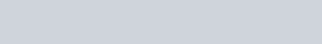Thermochemistry – JEE Advanced Previous Year Questions with Solutions
JEE Advanced Previous Year Questions of Chemistry with Solutions are available at eSaral. Practicing JEE Advanced Previous Year Papers Questions of Chemistry will help the JEE aspirants in realizing the question pattern as well as help in analyzing weak & strong areas.SimulatorPrevious Years JEE Advance Questions
Q. In a constant volume calorimeter, 3.5 g of a gas with molecular weight 28 was burnt in excess oxygen at 298.0 K. The temperature of the calorimeter was found to increase from 298.0 K to 298.45 K due to the combustion process. Given that the heat capacity of the calorimeter is 2.5 kJ$\mathrm{K}^{-1}$, the numerical value for the enthalpy of combustion of the gas in kJ $\mathrm{mol}^{-1}$ is [JEE 2009]

Download eSaral App for Video Lectures, Complete Revision, Study Material and much more...

Sol. 9$\mathrm{q}=(2.5)(0.45)=1.125$Q. Using the data provided, calculate the multiple bond energy $\left(\mathrm{kJ} \operatorname{mol}^{-1}\right)$ of a C C bond in $\mathrm{C}_{2} \mathrm{H}_{2}$. That energy is (take the bond energy of a C–H bond as 350 kJ $\left.\mathrm{mol}^{-1} .\right)$(A) 1165(B) 837(C) 865(D) 815 [JEE 2012]

Download eSaral App for Video Lectures, Complete Revision, Study Material and much more...

Sol. (D)